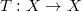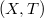ď»ż

### Introduzione

#### Abstract

En
We give a standard proof of the results of Tulipani: if$T:X \rightarrow X$ and a dicotomic family exisists on$(X,T)$ then a continuous finitely additive probability measure (charge),which is invariant for T, exisists.

DOI Code: §

Full Text: PDF